﻿ 湘江流域城市化与水资源环境交互耦合机制研究 Analysis of Interactive Coupling Mechanism between Urbanization and Water Resource Environment in the Xiangjiang River Basin

Journal of Water Resources Research
Vol.07 No.01(2018), Article ID:23011,13 pages
10.12677/JWRR.2018.71010

Analysis of Interactive Coupling Mechanism between Urbanization and Water Resource Environment in the Xiangjiang River Basin

Xingle Luo, Zhimei Jiang, Chujing Zheng, Jingbao Li*

College of Resources and Environment Science, Hunan Normal University, Changsha Hunan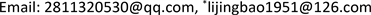Received: Nov. 25th, 2017; accepted: Dec. 5th, 2017; published: Dec. 13th, 2017ABSTRACT

Based on the comprehensive index system between urbanization and water resource & environment of the Xiangjiang River basin, a coupling coordination model and GRA (Grey Relational Analysis) model were established to analyze quantitatively space-time dynamics of urbanization and water resources environment by coupling coordination degree, and to reveal the interactive coupling mechanism of two systems. The results are: 1) In the last 15 years, the coupling coordination between urbanization and water resources & environment in the Xiangjiang River basin trends upward totally while the internal mechanism remains to be optimized. 2) The coordination degrees of these cities show characteristically spatial differentiation, which is related to the economic development and natural conditions. 3) The driving mechanism is extremely complicated. As a whole, the background of water resources is the main determinant of water resources and environment while the population structure is the main stressor of that. Economic urbanization is the main determinant of the urbanization level of a river basin while the water supply facilities and environmental pressure primarily constrain the process of urbanization. However, the driving mechanism of each city shows pertinent regional differences, which is closely related to the urban economic development model.

Keywords:Urbanization, Water Resource and Environment, Interactive Coupling, Sequential Variation, Xiangjiang River Basin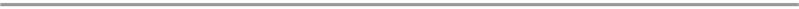1. 引言

2. 研究区域及方法

2.1. 研究区域概况Figure 1. Diagram of the Xiangjiang River district

2.2. 研究方法

2.2.1. 指标体系的建立

2.2.2. 数据来源与预处理

${{x}^{\prime }}_{ij}=\frac{{x}_{ij}-{m}_{ij}}{{M}_{ij}-{m}_{ij}}=\left\{\begin{array}{l}1\text{\hspace{0.17em}}\text{\hspace{0.17em}}\text{\hspace{0.17em}}\text{\hspace{0.17em}}\text{\hspace{0.17em}}\text{\hspace{0.17em}}\text{\hspace{0.17em}}\text{\hspace{0.17em}}\text{\hspace{0.17em}}\text{\hspace{0.17em}}\text{\hspace{0.17em}}\text{\hspace{0.17em}}\text{\hspace{0.17em}}\text{\hspace{0.17em}}\text{\hspace{0.17em}}\text{\hspace{0.17em}}\text{\hspace{0.17em}}\text{\hspace{0.17em}}\left({x}_{ij}\ge {M}_{ij}\right)\\ \frac{{x}_{ij}-{m}_{ij}}{{M}_{ij}-{m}_{ij}}\text{\hspace{0.17em}}\text{\hspace{0.17em}}\text{\hspace{0.17em}}\text{\hspace{0.17em}}\left({m}_{ij}\le {x}_{ij}\le {M}_{ij}\right)\\ 0\text{\hspace{0.17em}}\text{\hspace{0.17em}}\text{\hspace{0.17em}}\text{\hspace{0.17em}}\text{\hspace{0.17em}}\text{\hspace{0.17em}}\text{\hspace{0.17em}}\text{\hspace{0.17em}}\text{\hspace{0.17em}}\text{\hspace{0.17em}}\text{\hspace{0.17em}}\text{\hspace{0.17em}}\text{\hspace{0.17em}}\text{\hspace{0.17em}}\text{\hspace{0.17em}}\text{\hspace{0.17em}}\text{\hspace{0.17em}}\text{\hspace{0.17em}}\left({x}_{ij}\le {m}_{ij}\right)\end{array}$

${{x}^{\prime }}_{ij}=\frac{{M}_{ij}-{x}_{ij}}{{M}_{ij}-{m}_{ij}}=\left\{\begin{array}{l}1\text{\hspace{0.17em}}\text{\hspace{0.17em}}\text{\hspace{0.17em}}\text{\hspace{0.17em}}\text{\hspace{0.17em}}\text{\hspace{0.17em}}\text{\hspace{0.17em}}\text{\hspace{0.17em}}\text{\hspace{0.17em}}\text{\hspace{0.17em}}\text{\hspace{0.17em}}\text{\hspace{0.17em}}\text{\hspace{0.17em}}\text{\hspace{0.17em}}\text{\hspace{0.17em}}\text{\hspace{0.17em}}\text{\hspace{0.17em}}\text{\hspace{0.17em}}\text{\hspace{0.17em}}\text{\hspace{0.17em}}\text{\hspace{0.17em}}\left({x}_{ij}\le {m}_{ij}\right)\\ \frac{{M}_{ij}-{x}_{ij}}{{M}_{ij}-{m}_{ij}}\text{\hspace{0.17em}}\text{\hspace{0.17em}}\text{\hspace{0.17em}}\text{\hspace{0.17em}}\text{\hspace{0.17em}}\text{\hspace{0.17em}}\left({m}_{ij}\le {x}_{ij}\le {M}_{ij}\right)\\ 0\text{\hspace{0.17em}}\text{\hspace{0.17em}}\text{\hspace{0.17em}}\text{\hspace{0.17em}}\text{\hspace{0.17em}}\text{\hspace{0.17em}}\text{\hspace{0.17em}}\text{\hspace{0.17em}}\text{\hspace{0.17em}}\text{\hspace{0.17em}}\text{\hspace{0.17em}}\text{\hspace{0.17em}}\text{\hspace{0.17em}}\text{\hspace{0.17em}}\text{\hspace{0.17em}}\text{\hspace{0.17em}}\text{\hspace{0.17em}}\text{\hspace{0.17em}}\text{\hspace{0.17em}}\text{\hspace{0.17em}}\text{\hspace{0.17em}}\left({x}_{ij}\ge {m}_{ij}\right)\end{array}$ (1)Table 1. Coupling evaluation indicator system between urbanization system and water resource & environment system in Xiangjiang River Basin

2.2.3. 权重计算

${V}_{j}={S}_{j}/{\stackrel{¯}{X}}_{j}$

${\stackrel{¯}{X}}_{i}=\frac{1}{n}{\sum }_{i=1}^{n}{X}_{ij}$${S}_{j}=\sqrt{\frac{1}{n-1}{\sum }_{i=1}^{n}{\left({X}_{ij}-{\stackrel{¯}{X}}_{j}\right)}^{2}\text{\hspace{0.17em}}\left(j=1,2,3,\cdots ,20\right)}$ (2)

${\omega }_{i}={V}_{i}/{\sum }_{i=1}^{20}{V}_{i}$ (3)

${S}_{ij}={\omega }_{j}×{{x}^{\prime }}_{ij}$ (4)

${S}_{i}={\sum }_{i=1}^{n}{S}_{ij}$ (5)

2.2.4. 耦合协调度模型

${C}_{n}={\left[\frac{\left({u}_{1}×{u}_{2}×\cdots ×{u}_{1}\right)}{{\prod }^{\text{​}}\left({u}_{i}+{u}_{j}\right)}\right]}^{\frac{1}{2}}$ (6)

$T={\left(\alpha {U}_{1}+\beta {U}_{2}\right)}^{\frac{1}{2}}$ (7)

$D={\left(C×T\right)}^{\frac{1}{2}}$ (8)

2.2.5. 灰色关联度模型

${\sum }_{i=1}^{n}{\delta }_{ij}\left(t\right)=\frac{{\Delta }_{\mathrm{min}}+k{\Delta }_{\mathrm{max}}}{{\Delta }_{ij}\left(t\right)+k{\Delta }_{\mathrm{max}}}$ (9)

${\Delta }_{\mathrm{max}}=\mathrm{max}\mathrm{max}|{Z}_{i}^{x}\left(t\right)-{Z}_{j}^{y}\left(t\right)|$

${\Delta }_{\mathrm{min}}=\mathrm{min}\mathrm{min}|{Z}_{i}^{x}\left(t\right)-{Z}_{j}^{y}\left(t\right)|$ (10)

${Z}_{i}^{X}\left(t\right)$${Z}_{j}^{Y}\left(t\right)$ 分别表示长沙市t时刻城市化与水资源环境水平的标准化值，k为分表系数，一般取0.5。由于关系系数只代表各个时刻的关联程度，多且分散；为方便比较，采用求平均值的方法将各时刻关联系数集中在一个值上，其公式为：

${\gamma }_{ij}=\frac{1}{n}{\sum }_{i=1}^{n}{\delta }_{ij}\left(t\right)$ (11)

3. 测度结果与分析

3.1. 湘江流域城市化与水资源环境系统耦合协调度时序演变态势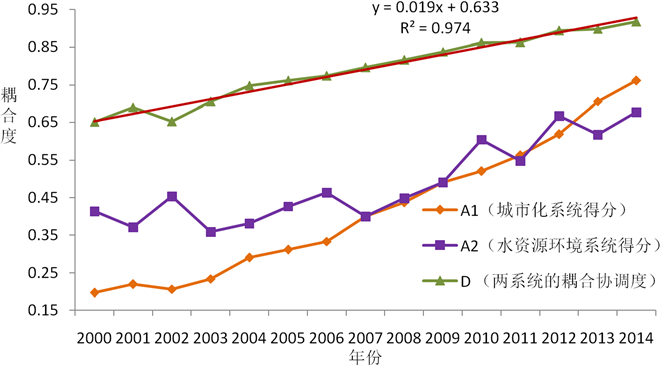Figure 2. The temporal dynamics of urbanization system, water resource & environment system and coupling coordination degree across the study area in 2000~2014

3.2. 湘江流域各城市空间组合及耦合协调特征

3.2.1. 湘江流域各城市耦合协调度空间分异及组合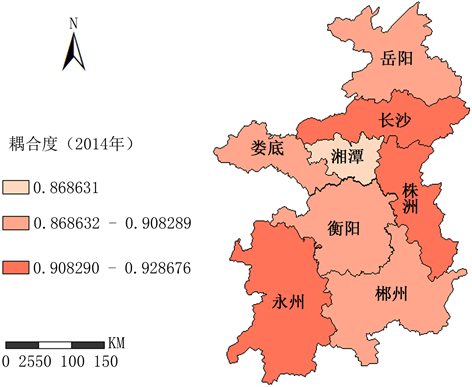Figure 3. Spatial distribution of coupling coordination degrees in the Xiangjiang River basin in 2014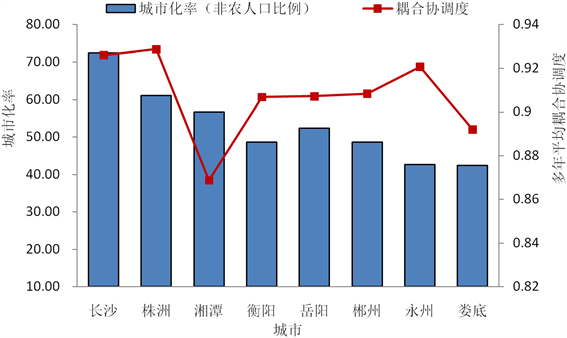Figure 4. Coupling coordination degree and urbanization rate of cities across the Xiangjiang River basin in 2014

3.2.2. 湘江流域各城市耦合协调度空间组合变化特征

1) 低城市化中耦合协调——娄底市。娄底市作为流域内发展历史较短的城市，城市化进程滞后。2014年娄底市人均GDP仅为流域平均水平的64%；经济结构落后，第三产业占GDP比重32% (流域平均水平36%)，为全流域最低；产业结构不协调、资源优势难以维继等因素限制了娄底经济社会的长足发展。水资源环境方面，娄底市有涟水、资水流经境内，加之降水充沛，水资源丰度大。此外，由于娄底市人均综合用水量相对较小，水资源环境负荷较小，城市化与水资源环境耦合关系处于中等协调状态。

2) 低城市化高耦合协调——永州市。由于地处湘江流域上游，地貌复杂、山地居多，基础设施不完善，永州市城市化水平相对较低(43%)，2014年人均GDP仅为全省平均水平的1/2。该市境内有湘江、珠江、资江三大河流，是全流域水资源丰度条件最好的市州。由于工业规模小(工业总产值为长沙的11.6%)，水资源年消耗量和污水排放量处于全流域最低水平，仅为长沙市的15.2%。加之城市规模较小、人均耗水量低，因此永州市城市化与水资源环境耦合关系呈高度协调状态。

3) 中城市化低耦合协调——湘潭市。该市建成区面积为全流域最小，受产业结构不合理等问题的制约，湘潭城市化水平为“长株潭”城市群的最低谷。其中心城区规模以上工业企业数量居全省第一，为株洲的2倍多，但利润不及株洲1/2。加之污水处理设施建设长期投入不足，污水处理厂处理能力仅为株洲的1/2。因此，湘潭市城市污水年排放总量大，仅次于长沙市，万元GDP污水排放量为全流域最高。经济发展模式落后导致水环境负荷过重，以致使城市化与水资源环境耦合关系呈低耦合协调的状态。

4) 中城市化中耦合协调——郴州、衡阳、岳阳。三市分别处于全流域上中下游地区，水资源本底条件优越，水利工程建设投资较高，大中小型水库数量仅次于永州市。郴州、衡阳、岳阳三市的环保产业单位数和收入均居全流域前列，污水处理率较高，水资源环境抗逆能力较高。因此岳阳、衡阳、郴州三市城市化与水资源环境耦合关系属中等协调。

5) 高城市化高耦合协调——长沙、株洲。长沙、株洲作为湖南经济发展和科技创新的龙头，城市化水平位居全省前列，二市水资源利用效率高，万元GDP用水量全流域最低水平。水资源环境治理能力强，污水处理率分居全流域一、二位；水资源保障能力强，城市供水综合生产能力和供水管道长度均居全流域一、二位。因此，长沙、株洲二市的城市化与水资源环境耦合关系处于高度协调水平。

3.3.湘江流域城市化与水资源环境的交互耦合的影响机制

3.3.1. 城市化与水资源环境系统之间的驱动因素

1) 制约城市化发展的主要因素

2) 胁迫水资源环境的主要因素

3.3.2. 城市化与水资源环境系统内驱动因素

1) 城市化发展综合水平

2) 水资源环境综合水平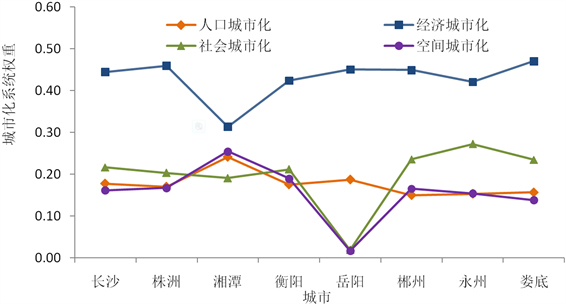Figure 5. Urbanization system weights of cities across the Xiangjiang River basin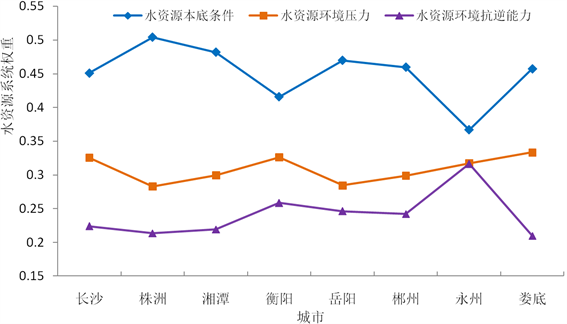Figure 6. Water resources and environment system weights of cities across the Xiangjiang River basin

4. 结论与讨论

1) 湘江流域2000~2014年城市化进程与水资源的协调关系整体趋向优化，但局部存在波动。在该背景下，研究区城市化与水资源环境耦合的内部机制有待进一步完善。

2) 湘江流域各市州城市化发展水平和耦合协调度具有明显的空间分异。根据人口城镇化水平和耦合协调度，将湘江流域各市州分为5种类型，并为流域内水资源调配和生态补偿的实施提供决策依据。

3) 湘江流域城市化与水资源环境耦合协调机制呈现出交互耦合的复杂关系。就系统内部指标而言，水资源环境的取决于水资源本底条件，受制于资源丰度和人均水平；经济城市化是湘江流域城市发展的主导因素，受工业规模和产业结构影响。就系统间指标而言，供水设施和环境压力是城市化发展的主要约束因素，人口结构是水资源环境的主要胁迫因素。在湘江流域一般机制下，各市州的驱动机制呈现出一定的地域差异。

2016年国家级大学生创新创业训练计划项目(201610542014)。

Analysis of Interactive Coupling Mechanism between Urbanization and Water Resource Environment in the Xiangjiang River Basin[J]. 水资源研究, 2018, 07(01): 84-96. http://dx.doi.org/10.12677/JWRR.2018.71010

1. 1. 方创琳, 孙心亮. 河西走廊水资源变化与城市化过程的耦合效应分析[J]. 资源科学, 2005, 27(2): 2-9. FANG Chuanglin, SUN Xinliang. Coupling effects between water resources change and urbanization process in Hexi Corridor of Northwest China. Resources Science, 2005, 27(2): 2-9. (in Chinese)

2. 2. LETTENMAIER, D. P., WOOD, A. W., PALMER, R. N., et al. Water resources implications of global warming: A U.S. regional perspective. Climatic Change, 1999, 43(3): 537-579.

3. 3. VÖRÖSMARTY, C. J., GREEN, P., SALISBURY, J., et al. Global water resources: Vulnerability from climate change and population growth. Science, 2000, 289(5477): 284-288.

4. 4. RHODES, A. L., NEWTON, R. M. and PUFALL, A. Influences of land use on water quality of a diverse New England watershed. Environmental Science & Technology, 2001, 35(18): 3640.

5. 5. 方创琳, 黄金川, 步伟娜. 西北干旱区水资源约束下城市化过程及生态效应研究的理论探讨[J]. 干旱区地理, 2004, 27(1): 1-7. FANG Chuanglin, HUANG Jinchuan and BU Weina. Theoretical study on urbanization process and ecological effect with the restriction of water resource in arid area of northwest China. Arid Land Geography, 2004, 27(1): 1-7. (in Chinese)

6. 6. 刘耀彬, 李仁东, 宋学锋. 中国区域城市化与生态环境耦合的关联分析[J]. 地理学报, 2005, 60(2): 237-247. LIU Yaobin, LI Rendong and SONG Xuefeng. Grey associative analysis of regional urbanization and eco-environment coupling in China. Acta Geographica Sinica, 2005, 60(2): 237-247. (in Chinese)

7. 7. ALCAMO, J., FLÖRKE, M. and MÄRKER. M. Future long-term changes in global water resources driven by socio-economic and climatic changes. Hydrological Sciences Journal, 2007, 52(2): 247-275.

8. 8. DESSU, S. B., MELESSE, A. M., BHAT, M. G., et al. Assessment of water resources availability and demand in the Mara River Basin. Catena, 2014, 115(3): 104-114.

9. 9. 汪慧贞, 李宪法. 北京城区雨水径流的污染及控制[J]. 城市环境与城市生态, 2002, 15(2): 16-18. WANG Huizhen, LI Xianfa. Pollution and control of stormwater runoff in Beijing urban area. Urban Environment and Urban Ecology, 2002, 15(2): 16-18. (in Chinese)

10. 10. 曾晓燕, 牟瑞芳, 许顺国. 城市化对区域水资源的影响[J]. 资源环境与工程, 2005, 19(4): 318-322. ZENG Xiaoyan, MOU Ruifang and XU Shunguo. The influence of urbanization on regional water resources. Resources; Environment and Engineering, 2005, 19(4): 318-322. (in Chinese)

11. 11. 贾绍凤, 张士锋, 杨红. 工业用水与经济发展的关系[J]. 自然资源学报, 2004, 19(3): 2799-2284. JIA Shaofeng, ZHANG Shifeng and YANG Hong. Relationship between industrial water use and economic development. Journal of Natural Resources, 2004, 19(3): 2799-2284. (in Chinese)

12. 12. 董林, 陈璇璇. 城市可持续发展的水资源约束分析[J]. 水利科技与经济, 2006, 12(8): 525-527. DONG Lin, CHEN Xuanxuan. Water resources constraint analysis of urban sustainable development. Water Conservancy Science and Technology and Economy, 2006, 12(8): 525-527. (in Chinese)

13. 13. 湖南省统计局. 湖南统计年鉴(2006-2014) [M]. 北京:中国统计出版社, 2014. Hunan Provincial Bureau of Statistics. Hunan statistical yearbook (2006-2014). Beijing: China Statistics Press, 2006-2014. (in Chinese)

14. 14. 康慕谊. 城市生态学与城市环境[M]. 北京: 中国计量出版社, 1997: 345-346. KANG Muyi. City ecology and city environment. Beijing: Chinese Metrology Press, 1997: 345-346. (in Chinese)

15. 15. FAO Proceedings. Land quality indicators and their use in sustainable agriculture and rural development. Proceedings of the Workshop Organized by the Land and Water Development Division FAO Agriculture Department, 1997, 2: 5-5.

16. 16. WALZ, R. Development of environmental indicator systems: Experiences from Germany. Environmental Management, 2000, 25(6): 613-623. https://doi.org/10.1007/s002670010048

17. 17. 李静芝, 朱翔, 李景保, 等. 洞庭湖区城镇化进程与水资源利用的关系[J]. 应用生态学报, 2013(6): 1677-1685. LI Jingzhi, ZHU Xiang, LI Jingbao, et al. The relationship between urbanization process and water resources utilization in Dongting Lake area. Chinese Journal of Applied Ecology, 2013(6): 1677-1685. (in Chinese)

18. 18. 庄楚强, 吴亚森. 应用数理统计基础[M]. 广州: 华南理工大学出版社, 2004. ZHUANG Chuqiang, WU Yasen. Application of mathematical statistics basis. Guangzhou: South China University of Technology Press, 2004. (in Chinese)

19. 19. SHAW, P. J. A. Multivariate statistics for the Environment Sciences. A Hodder Arnold Publication, 2003: 38.

20. 20. 邓聚龙. 社会经济灰色系统的理论与方法[J]. 中国社会科学, 1984(6): 47-60. DENG Julong. Theory and method of socio economic grey system. Chinese Social Sciences, 1984(6): 47-60. (in Chinese)